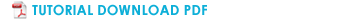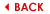# TUTORIALS > ADDING EXTERNAL FUNCIONALITIES TO IMAGELAB

The language development of plugins for ImageLab is the C + + and ambinete suggested for developing / using custom filters is Linux.

To follow this tutorial, you will need to have installed the following packages:

cmake
ccmake
g++
gcc
libqt4-dev
libqt4-gui
libqtcore4

VTK:

To develop a filter plugin, you must have a version of VTK is compiled and source code available.

Install the package libvtk5-dev (Ubuntu: Go to “System-Administration” and start “Synaptic” and select the package) or download the source and compile VTK (do not use version 5.6.1):

http://www.vtk.org/files/release/5.4/vtkdata-5.4.2.tar.gz
tar -zxvf vtk-5.4.2.tar.gz
mkdir VTK_build
cd VTK_build
ccmake ../VTK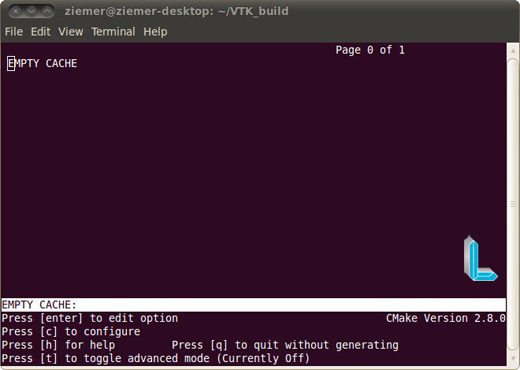Press the letter C (“Press [c] to configure”) until the following screen options appear: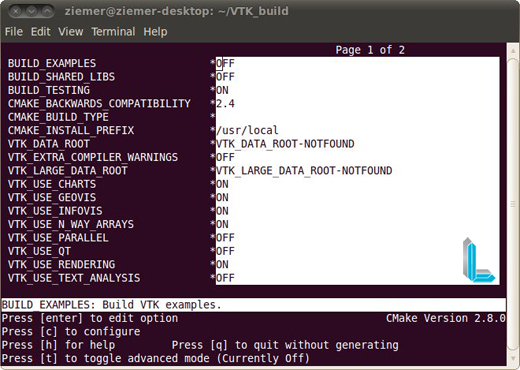After, you need to change the value of some compilation variables: place the cursor over the option and enable editing of the value by pressing “enter”:

BUILD_EXAMPLES OFF
BUILD_SHARED_LIBS ON
BUILD_TESTING OFF

After, you need to change the value of some compilation variables: place the cursor over the option and enable editing of the value by pressing “enter”.

Then, press the letter “g” to generate makefiles (the control will return to the terminal command)

To compile the VTK: make or make-j2 (compilation generates two threads – the optimal number of threads is based on the number of process colors).

Depending on the configuration of the machine, the compilation may take a while.

Programming the filter Plugin

Download the package with the auxiliary files to build the filter plugin:

http://hemo01a.lncc.br/software/ImageLab_dev/imPluginFilter.tar.gz

In this file there are implementations of the following classes:

imPluginBaseFilter: class “father” of the class that implements the filter plugin;

imPluginFilter: class in which the code that manipulates the pixels to be described – Update method.

This class contains a reference to an object of type vtkImageData (this-> image) that is the image itself.

Other class methods vtkImageData:
http://www.vtk.org/doc/nightly/html/classvtkImageData.html

Commands to compile the filter plugin:

cd imPluginFilter
mkdir build
cd build
ccmake ../

Within the environment of the CCMAKE, manually point the directory path where the compiled VTK (example: home / user / VTK / BUILD) VTK_DIR the variable – if the VTK has been installed packet, the path will be filled automatically.

Generate the makefiles (using the “g”).

Compile the library containing the objects of the filter plugin with the command make.

A library is generated with the following name: libImageLabPluginFilter.so

To incorporate the library in ImageLab plugin, type the following command before starting the ImageLab

export LD_LIBRARY_PATH=\$LD_LIBRARY_PATH:/path

Path is the path where the generated file. Is located so.

Methods to be used in programming the filter:

Basically, the parameters are passed in vectors of integers and real values for the filter.

Inside the filter class, access to parameters is done through the following methods:

Returns reference to an array of type double with three positions (x, y, z) containing the coordinates of a given seed – returns NULL if the seed is not found:
double *GetSeed(int seedNumber);

Returns the value of a pixel assigned to a particular seed:
double GetSeedPixelValue(int seedNumber);

Returns the integer parameter (defined in the filter plugin interface) with index given by the parameter paramNumber:
int GetIntParam(int paramNumber);

Returns the actual parameter (defined in the filter plugin interface) with index given by the parameter paramNumber:
double GetDoubleParam(int paramNumber);

Interface filter plugin:

The interface is pretty generic and it is intended that in future versions, in addition to the code, the user can also specify interfaces.
In the figure below, one can see that there are basically three parameter fields:
The first field is for integer parameters (these must be separated by a semicolon);
The second field is for real and these parameters should also be separated by a semicolon;
The last field specifies the seeds (seeds) “placed” in the image.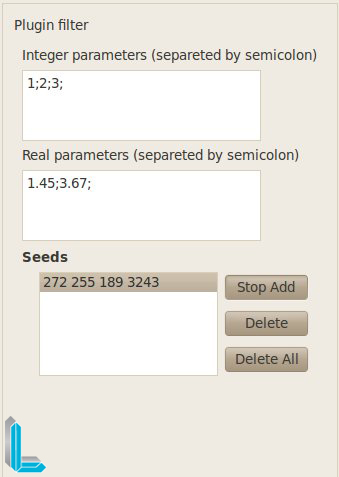Examples of codes

Example 1:”Hello World”

```cout << "Image with the following characteristics: " << endl;
cout << "Number of dimensions of the image "<< image->GetDataDimension() << endl;

double* spacing = image->GetSpacing();

cout << "width -x: "<< spacing << endl;
cout << "height -y: "<< spacing << endl;
cout << "length -z: "<< spacing << endl;

int* dims = image->GetDimensions(); // get the size of the image array, x, y, z

cout << "planos em x: "<< dims << endl;
cout << "planos em y: "<< dims << endl;
cout << "planos em z: "<< dims << endl;```

Example 2: Threshold in terms of pixel value obtained from a seed

double PixelValue = this->GetSeedPixelValue(0);

cout << “Value associated with pixel Seed 0 “<< PixelValue << endl;

double limiteInf= PixelValue; // value obtained from the interface – that lower values are reset

cout << “Pixel values that are less than “ << limiteInf << ” will be cleared ” <<< endl;

for (int y=0; y<dims; y++)

{

for (int x=0; x<dims; x++)

{

for (int z=0; z<dims; z++)

{

double CurrentPixelValue = image->GetScalarComponentAsDouble(x,y,z,0);

// Comparing the current pixel with the first real value

// Placed at the interface of real parameters

if (CurrentPixelValue > limiteInf)

image->SetScalarComponentFromDouble(x,y,z,0,CurrentPixelValue);

else

image->SetScalarComponentFromDouble(x,y,z,0,0.0);

}

}

}